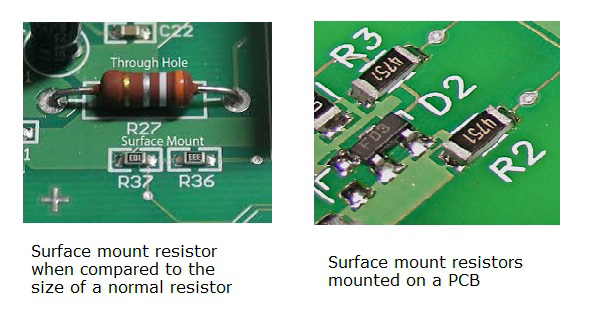# Basic Electronics - Non linear Resistors

There are many types of resistors according to the type of material used, the manufacturing procedure and their applications. The classification is as shown below.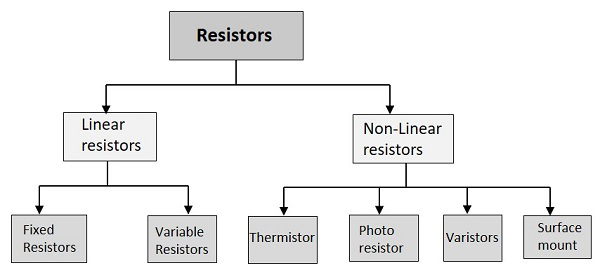Linear resistors have linear VI characteristics and non-linear resistors has non-linear VI characteristics. Non-linear resistors are the resistors whose voltage and current characteristics vary non-linearly. The voltage and current values vary depending upon other factors like temperature and light, but they may not be linear.

## Thermistor

Thermal means temperature. In this resistor, the resistance varies with temperature. If heat increases, the resistance decreases and vice versa. This is used for measurement and control purposes.

The main types of thermistors are NTC and PTC.

• NTC is Negative Temperature Coefficient and in such devices, the resistance decreases as the temperature increases. These are used to protect the devices from over-voltage conditions.

• PTC is Positive Temperature Coefficient and in such devices, the resistance increases as the temperature increases. These are used to protect the devices from over current conditions.

The following figure shows an NTC thermistor, along with its symbol.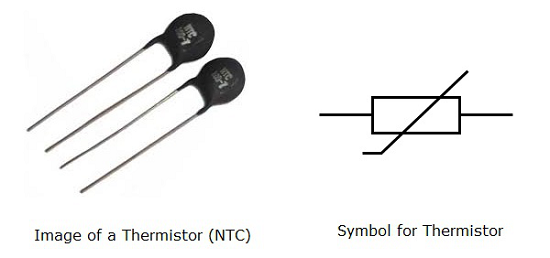## Photo Resistor

Photo means light. In this resistor, the resistance varies with light. As light increases resistance decreases and vice versa. This is also used for measurement and control purposes. It is also called as LDR (Light Dependent Resistor)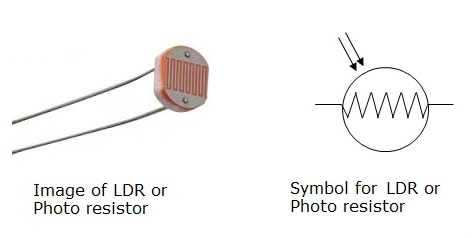## Varistors

The resistance of a varistor, varies with the applied voltage. As the voltage increases, the resistance decreases and if the voltage decreases, the resistance increases. It is also called as VDR (Voltage Dependent Resistor).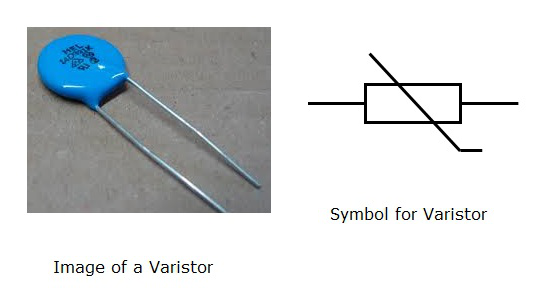## Surface Mount

These are being highly used since the introduction of surface mount technology. These can be termed as chip resistors, which means a resistive layer integrated on a ceramic chip.

These surface mount resistors are very small when compared to the normal resistors and hence occupy less space. They are effective and dissipate less heat. The invention of these resistors has changed the look of a PCB (Printed Circuit Board) and reduced its size greatly.

The advantages of surface mount resistors are −

• These are compact in size.
• These are very stable.
• They have good tolerance.
• They are effective in reducing heat dissipation.

The following figure shows the images of surface mount resistors.